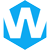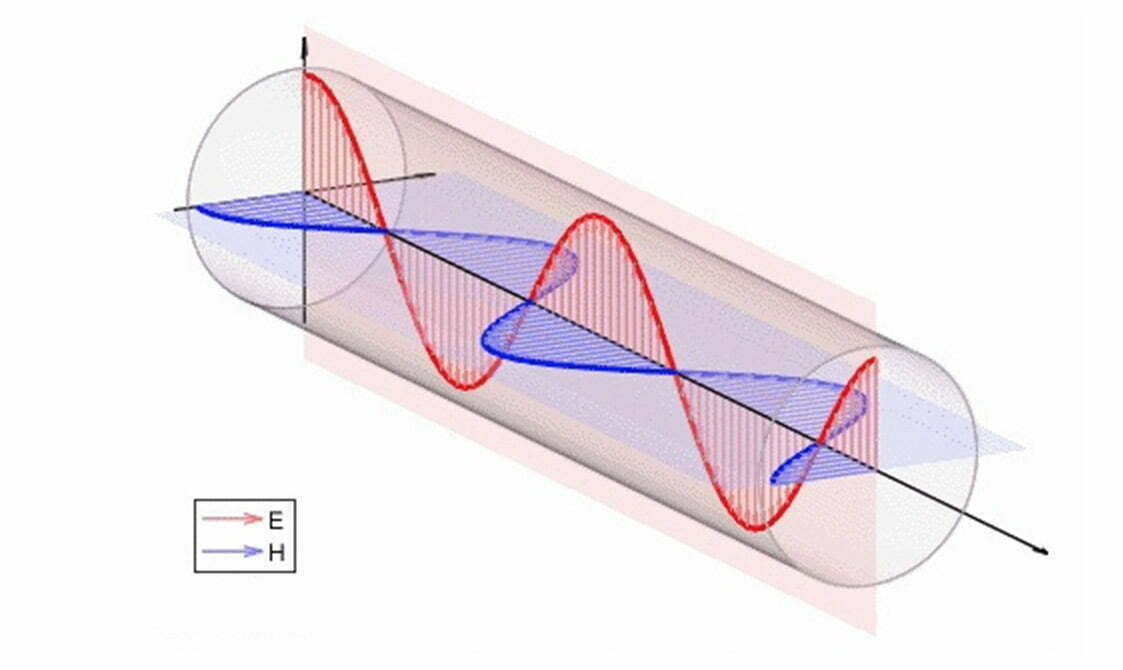# Definition of Electromagnetic Waves## What Are Electromagnetic Waves?

The electromagnetic wave is a kind of energy, which belongs to a kind of wave, just like mechanical wave, gravitational wave and matter wave (probability wave). The higher the frequency, the higher the frequency of the emitted electromagnetic wave and the shorter the wavelength. This electromagnetic wave is called black body radiation. Just as people have been living in the air but eyes cannot see the air, in addition to light waves, people cannot see other electromagnetic waves that are ubiquitous.

## How do electromagnetic waves occur?

The electromagnetic field includes two aspects of electric field and magnetic field, and its characteristics are represented by electric field strength E (or electric displacement D) and magnetic flux density B (or magnetic field strength H) respectively.

According to Maxwell’s electromagnetic field theory, these two parts are closely related. A time-varying electric field causes a magnetic field, and a time-varying magnetic field causes an electric field.

When the field source of the electromagnetic field changes with time, the electric field and the magnetic field excite each other to cause the movement of the electromagnetic field to form electromagnetic waves. The propagation speed of electromagnetic waves is equal to the speed of light. In free space, it is c=299792458m/s≈3×108m/s. The travel of electromagnetic waves is also accompanied by the delivery of power.

## Properties of Electromagnetic Waves

Electromagnetic waves have three properties, namely amplitude (intensity, light intensity), frequency (wavelength) and waveform (spectral distribution). For visible light, these three correspond to the lightness, hue and chromaticity of light color respectively. As far as the electromagnetic wave is concerned, there is also the concept of the initial phase. Its waveform is a sine curve (cosine curve), called a sine wave (cosine wave). The closer the waveform of an electromagnetic wave is to a sine wave, the purer its spectrum and the better its monochromaticity. Typical An example of this is lasers.

### 1. Electromagnetic Amplitude

The maximum absolute value that the electric field strength (E) and magnetic field strength (H) of the electromagnetic wave can achieve is called the maximum value or amplitude of the electromagnetic wave. The amplitude of electromagnetic waves is divided into electric field amplitude and magnetic field amplitude, and the units are V/m and A/m respectively. They can all represent the intensity of electromagnetic waves, but in practical applications, electric field strength is more often used as the representation method of electromagnetic wave amplitude.

#### 1.1 Electric field strength

The electric field strength is a physical quantity used to express the strength and direction of the electric field, and it is often expressed by “E”.

##### 1.11 Calculation formula

In different cases, the calculation method of electric field strength is different. The following formula is only applicable to point charges:

E=KQ/r2

The “E” is the electric field strength, “K” is the electrostatic force constant, “Q” is the magnitude of the source charge, and “r” is the distance between the source charge and the test charge.

#### 1.2 magnetic field intensity

Magnetic field strength was first derived from the viewpoint of magnetic charge in history. Analogous to Coulomb’s law of charge, it is believed that there are two kinds of magnetic charges, positive and negative, in nature, and Coulomb’s law of magnetic charge is proposed. The force experienced by a unit positive electromagnetic charge in a magnetic field is called the magnetic field strength (symbol H).

##### 1.21 Calculation formula

In a medium, the formula for calculating the magnetic field strength is:

H=B/μ0-M

B” is the magnetic induction, “M” is the magnetization, “μ0” is the magnetic permeability in vacuum, μ0=4π×10-7 Tesla m/A. The unit of “H” is An/meter.

### 2 Electromagnetic Frequency

The number of electromagnetic fluctuations per second is called electromagnetic frequency.

#### Calculation formula

The formula for calculating the frequency of electromagnetic waves is:

f(Hz)=c(m/s)(m)

c“: wave speed (the speed of light is a constant, equal to 299792458m/s in a vacuum, approximately equal to 3×108m/s) unit: m/s;
f“: frequency (unit: Hz, 1MHz=1000kHz=1×106Hz);
λ“: wavelength (unit: m);

### 3 Electromagnetic Spectrum

Arranging these electromagnetic waves in the order of wavelength or frequency is the electromagnetic spectrum. If the frequencies of each band are arranged in order from low to high, they are power frequency electromagnetic waves, radio waves (divided into long waves, medium waves, short waves, microwaves), infrared rays, visible light, ultraviolet rays, X-rays and gamma rays. Radio has the longest wavelength, and cosmic rays (X-rays, gamma rays, and rays with shorter wavelengths) have the shortest wavelengths.

## Applications of Electromagnetic Waves

• Radio waves: for communication, etc;
• Microwave: used in microwave ovens, satellite communications, etc;
• Infrared rays: used for remote control, thermal imagers, infrared guided missiles, etc;
• Visible light: It is the basis for all living things to see things;
• Ultraviolet light: used for medical disinfection, verifying counterfeit banknotes, measuring distance, flaw detection in engineering, etc;
• X-ray: for CT photography;
Gamma rays: used for treatment, making atoms jump to produce new rays, etc.

#### Share:Aidan Taylor

I am Aidan Taylor and I have over 10 years of experience in the field of PCB Reverse Engineering, PCB design and IC Unlock.

## Recommended Post

### Need Help?

Don't hesitate - Get in touch today to find out how we can help take your project to the next level.

Scroll to Top## Start to Achieve Your PCB Project

Hello 👋
Can we help you? Chat with us on WhatsApp↓, or send an email to us: info@reversepcb.com